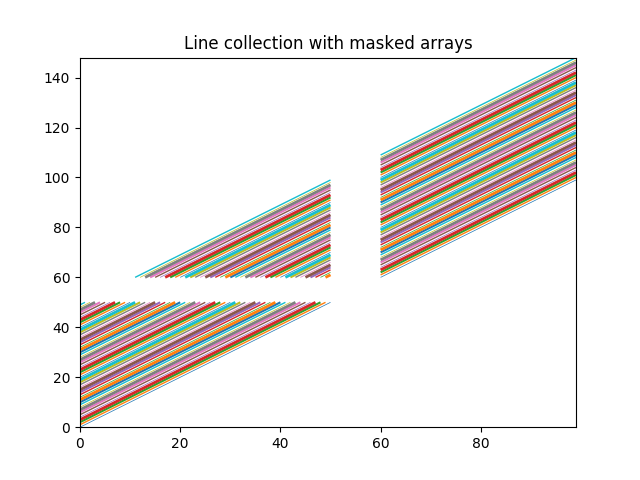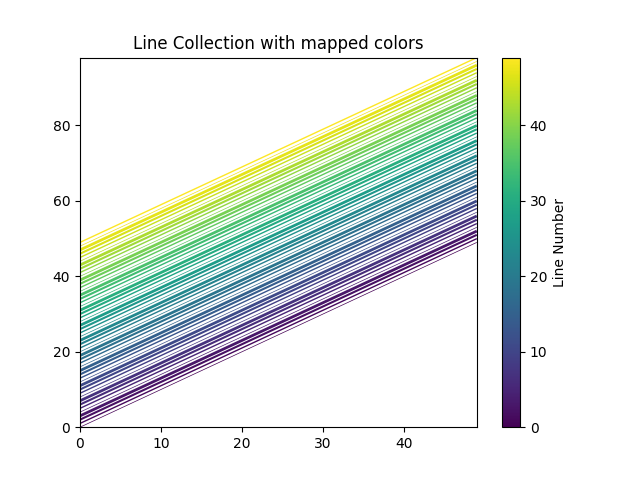Travis-CI:Line Collection¶

Plotting lines with Matplotlib.

matplotlib.collections.LineCollection allows one to plot multiple lines on a figure. Below we show off some of its properties.

import matplotlib.pyplot as plt
from matplotlib.collections import LineCollection
from matplotlib import colors as mcolors

import numpy as np

# In order to efficiently plot many lines in a single set of axes,
# Matplotlib has the ability to add the lines all at once. Here is a
# simple example showing how it is done.

x = np.arange(100)
# Here are many sets of y to plot vs x
ys = x[:50, np.newaxis] + x[np.newaxis, :]

segs = np.zeros((50, 100, 2), float)
segs[:, :, 1] = ys
segs[:, :, 0] = x

segs = np.ma.masked_where((segs > 50) & (segs < 60), segs)

# We need to set the plot limits.
fig, ax = plt.subplots()
ax.set_xlim(x.min(), x.max())
ax.set_ylim(ys.min(), ys.max())

# colors is sequence of rgba tuples
# linestyle is a string or dash tuple. Legal string values are
#          solid|dashed|dashdot|dotted.  The dash tuple is (offset, onoffseq)
#          where onoffseq is an even length tuple of on and off ink in points.
#          If linestyle is omitted, 'solid' is used
colors = [mcolors.to_rgba(c)
for c in plt.rcParams['axes.prop_cycle'].by_key()['color']]

line_segments = LineCollection(segs, linewidths=(0.5, 1, 1.5, 2),
colors=colors, linestyle='solid')
plt.show()In order to efficiently plot many lines in a single set of axes, Matplotlib has the ability to add the lines all at once. Here is a simple example showing how it is done.

N = 50
x = np.arange(N)
# Here are many sets of y to plot vs x
ys = [x + i for i in x]

# We need to set the plot limits, they will not autoscale
fig, ax = plt.subplots()
ax.set_xlim(np.min(x), np.max(x))
ax.set_ylim(np.min(ys), np.max(ys))

# colors is sequence of rgba tuples
# linestyle is a string or dash tuple. Legal string values are
#          solid|dashed|dashdot|dotted.  The dash tuple is (offset, onoffseq)
#          where onoffseq is an even length tuple of on and off ink in points.
#          If linestyle is omitted, 'solid' is used

# Make a sequence of x,y pairs
line_segments = LineCollection([list(zip(x, y)) for y in ys],
linewidths=(0.5, 1, 1.5, 2),
linestyles='solid')
line_segments.set_array(x)